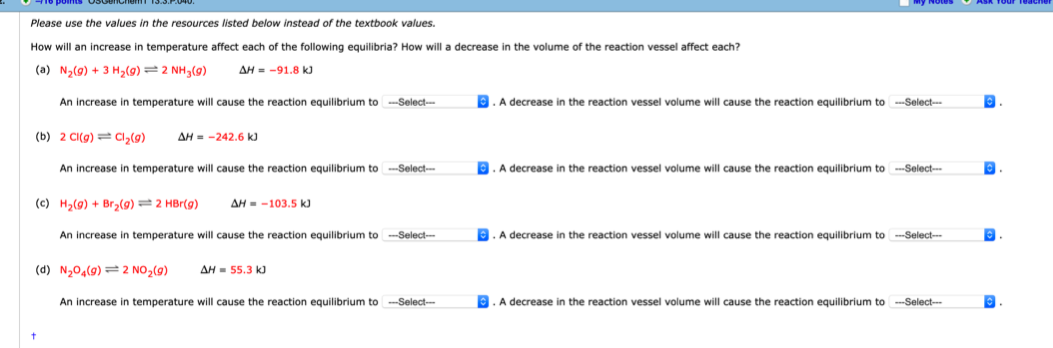# Please use the values in the resources listed below instead of the textbook values. How will an increase in temperature affect each of the following equilibria? How will a decrease in the volume of the reaction vessel affect each? (a) N2(g) + 3 H2(g) ‘=' 2 NH3(g) OH = —91.8 kJ An increase in temperature will cause the reaction equilibrium to_____. A decrease in the reaction vessel volume will cause the reaction equilibrium to _____ (b) 2 CI(g) ‘=' Cl2(g) OH = —242.6 kJ An increase in temperature will cause the reaction equilibrium to _____ A decrease in the reaction vessel volume will cause the reaction equilibrium to _____ (c) H2(g) + Br2(g) 2 HBr(g) OH = —103.5 kJ An increase in temperature will cause the reaction equilibrium to _____. A decrease in the reaction vessel volume will cause the reaction equilibrium to _____ (d) N204(g) = 2 NO2(g) LH = 55.3 kJ An increase in temperature will cause the reaction equilibrium to ____. A decrease in the reaction vessel volume will cause the reaction equilibrium to _____